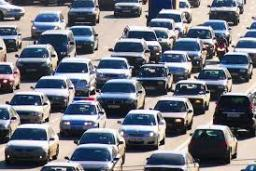# Average 4458

The first third of the track was driven by a car at a speed of 15 km/h, the second third at a speed of 30 km/h, and the last third at a speed of 90 km/h. Find the average speed of the car.

v =  27 km/h

### Step-by-step explanation:Did you find an error or inaccuracy? Feel free to write us. Thank you!

Tips for related online calculators
Looking for help with calculating arithmetic mean?
Looking for calculator of harmonic mean?
Looking for a statistical calculator?
Do you want to convert velocity (speed) units?

#### Grade of the word problem:

We encourage you to watch this tutorial video on this math problem: